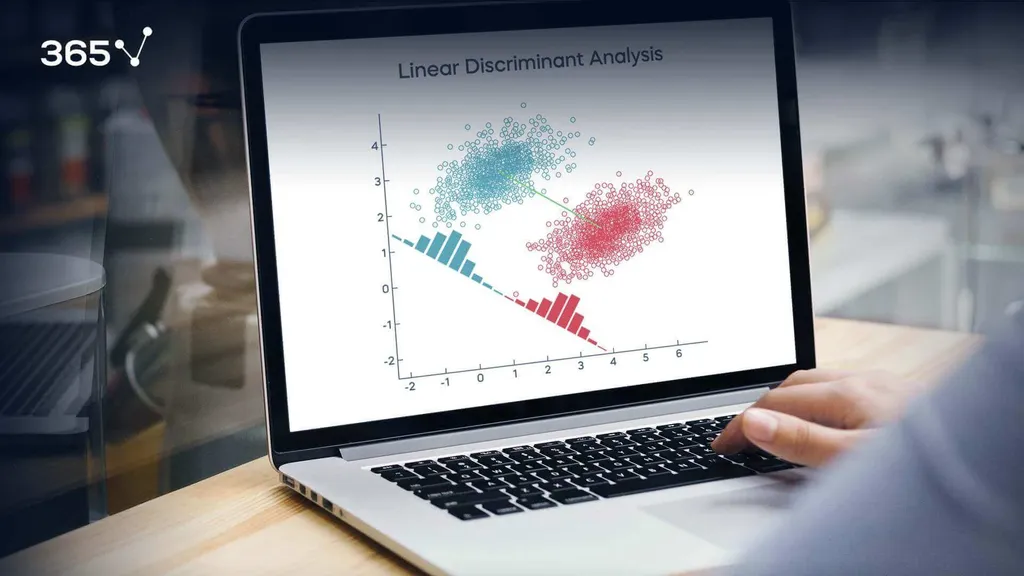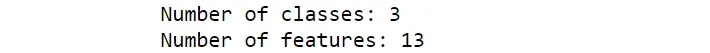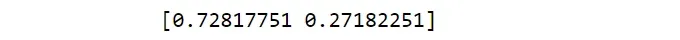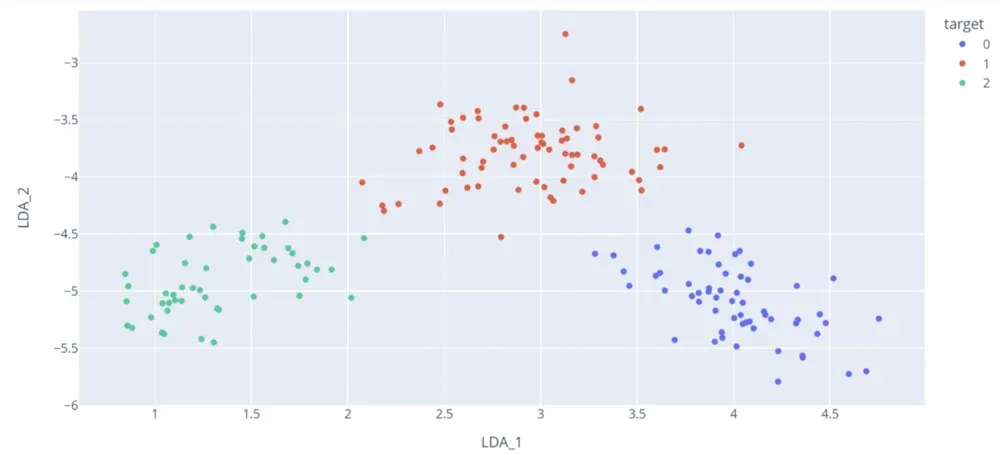# How to Perform LDA in Python with sk-learn?When working with huge amounts of data, there are often a lot of features available. The higher the number of variables, the bigger the risk of redundant information. This leads to the exigence of reducing the dataset’s dimensionality into a lower-dimensional space, called dimensionality reduction.

An approach that data scientists take when dealing with this issue is implementing linear discriminant analysis. It’s a popular choice amongst professionals due to its beneficial features, such as simplicity and low time complexity.

In general, linear algebra is quite useful for the data science workflow. If you’re looking to start a career in the industry and gain an edge over your peers, it’s a good idea to get acquainted with this branch of mathematics and LDA especially.

In this tutorial, we are going to provide an easy explanation of what LDA is, how it works and why you should use it. In addition, we will show you how to perform linear discriminant analysis from scratch in Python using sk-learn.

## What is Linear Discriminant Analysis?

Linear discriminant analysis, or LDA for short, is a supervised learning technique used for dimensionality reduction. It’s also commonly used as preprocessing step for classification tasks. The goal is to project the original data on a lower-dimensional space while optimizing the separability between different categories. In other words, LDA aims to find new axes that maximize the space between classes, while at the same time making each class as compact as possible.

There are strong assumptions behind its decisions. First of all, linear discriminant analysis assumes that all the categories are linearly separable by drawing a decision region between different classes. Moreover, the data corresponding to each class is assumed normal with the same covariance and different means.

For example, if we have two classes, LDA projects the data into this hyperplane in such a way that it maximizes the distance between the means of the different classes – this is called between-class variance. In the meantime, it minimizes the distance between the mean and the samples of each class, otherwise known as within-class variance.

We’ll see both matrices and how they’re computed later in the implementation.

## How Does Linear Discriminant Analysis Work?

Before moving on to the Python example, we first need to know how LDA actually works. The procedure can be divided into 6 steps:

1. Calculate the between-class variance. This is how we make sure that there is maximum distance between each class.
2. Calculate the within-class variance. This matrix helps us minimize the variance of the classes, thereby making each class as compact as possible.
3. Compute the eigenvectors and the corresponding eigenvalues. More specifically, those produced by the multiplication between the inverse within scatter matrix with the between scatter matrix. Without going into too much detail here, the eigenvalues and eigenvectors show the direction which can give us the maximum class separability based on the data.
4. Put the eigenvalues in decreasing order and select k eigenvectors with the largest eigenvalues. The eigenvectors with the largest eigenvalues show the most important features – that’s why they’re arranged in decreasing order.
5. Create a k dimensional matrix containing the eigenvectors. As a final step this matrix is then multiplied with the original, resulting in the new features from LDA.

## Why Use Linear Discriminant Analysis?

At this point, you are probably wondering why you need to apply linear discriminant analysis. Well, it can be useful for 2 different motivations:

### Dimensionality Reduction

The primary reason for applying LDA is for data compression. In particular, image compression, which is fundamental for taking up less memory space and capturing most of the information.

Recent applications have also emerged in medical imaging.

### Data Visualization

Another purpose for LDA is data visualization. Most of the time, the dataset has a huge number of features and it’s hard to summarize all the information in a unique plot. Projecting the data into 2 or 3 dimensions allows us to visualize it and, thus, understand the underlying logic.

## How to Perform Linear Discriminant Analysis in Python?

Here, you’ll see a step-by-step process of how to perform LDA in Python, using the sk-learn library. For the purposes of this tutorial, we’ll rely on the wine quality dataset, which contains measurements taken for different constituents found in 3 types of wine.

Let’s import the libraries and the dataset:

import pandas as pd
import numpy as np
import matplotlib.pyplot as plt
import plotly.express as px

df = pd.DataFrame(wine.data, columns=wine.feature_names)
df['target'] = wine.target


Before starting to calculate any quantities, let’s define the inputs X and target y variables.  Then, we must fix the number of samples, the number of features, and the number of components, which should respect a specific condition:

X = wine.data.astype('float32')
y = wine.target
n_samples, n_features = X.shape
classes = np.unique(y)
n_classes = len(classes)
n_components = 2
max_components = min(n_classes-1,n_features)
print("Number of classes: {}".format(n_classes))
print("Number of features: {}".format(n_features))
if n_components > max_components:
raise ValueError("the number of components cannot be larger than min(n_features,n_classes-1)")I want to point out that the number of components cannot be larger than the minimum between the number of features and the number of classes minus 1. Indeed, if we have 2 classes, then there is only a hyperplane separating the categories. Three classes can have a maximum of 2 components and so on.

Now, it’s time to calculate the between-class and within-class variance.

As explained before, the between-class variance represents the distance between the different classes. Thus, it takes into account the differences between the feature values of the specific class and the mean of all the observations.

On the other hand, the within-class variance is based on the difference between the feature values and the mean of each class.

These are the following formulas to calculate the two quantities, $S_W$ and $S_B$:

$S_{W} = \textstyle \sum _{c=1}^{C} S_{c}$
$S_{C} = \textstyle \sum _{i=1}^{n_{c}} (x{_{i}} - \overline{x}_{c}) (x{_{i}} - \overline{x}_{c})^{T}$
$S_{B} = \textstyle \sum _{c=1}^{C}(\overline{x}_{c} - \overline{x})^{T}$

Let’s calculate the between-class variance and the within-class variance with the following lines of code:

mean = np.mean(X,axis=0)
Sw = np.zeros((n_features,n_features))
Sb = np.zeros((n_features,n_features))
for c in classes:
Xc = X[y==c]
class_means = np.mean(Xc,axis=0)
#within-class variance
Sw += (Xc-class_means).T.dot(Xc-class_means)
mean_diff = (class_means-mean).reshape(n_features,1)
#between-class variance
Sb += n_classes * (mean_diff).dot(mean_diff.T)

Once we’ve obtained the 2 crucial matrices, we can finally compute the eigenvectors and the corresponding eigenvalues:

A = np.linalg.inv(Sw).dot(Sb)
eigen_values, eigen_vectors = np.linalg.eig(A)
eigen_vectors = eigen_vectors.T

We can arrange the eigenvalues in decreasing order and, later, select k eigenvectors with the largest eigenvalues. In this case, we’ll go with k=2, which corresponds to the number of components we want to select:

sorted_idxs = np.argsort(abs(eigen_values))[::-1]
eigen_values,eigen_vectors = eigen_values[sorted_idxs],eigen_vectors[sorted_idxs]
linear_discriminants = eigen_vectors[0:n_components]

Let’s also obtain the explained variance ratio from each component. It indicates the amount of variance each component of LDA holds after projecting the original data into the two-dimensional space:

explained_variance_ratio = np.sort(eigen_values / np.sum(eigen_values))[::-1][:max_components]
print(explained_variance_ratio)As a result, we learn that the first component of LDA captures approximately 73% of the variability between the categories, whereas the second component – only 27%. It makes sense since the new axes are ranked in order of importance and, consequently, the first component accounts for most of the variation.

Finally, we can compute the new features by multiplying the k dimensional matrix obtained in the previous step with the original data matrix:

X_lda = np.dot(X,linear_discriminants.T)
X_lda_df = pd.DataFrame({'LDA_1':X_lda[:,0],'LDA_2':X_lda[:,1]})
X_lda_df['target'] = y
X_lda_df['target'] = X_lda_df['target'].apply(lambda y: str(y))
Let’s visualize the wine dataset in a two-dimensional space:
fig = px.scatter(X_lda_df, x='LDA_1', y='LDA_2', color=X_lda_df.target,labels={'0': 'LDA 1', '1': 'LDA 2'})
fig.show()Et voilà! Now, the scatter plot can provide us with a good summary of the data’s information. We can clearly distinguish 3 clusters that correspond to different categories and there is no overlapping between the classes. Moreover, the points belonging to the same type of wine are very close to each other.

## Linear Discriminant Analysis in Python: Next Steps

Linear discriminant analysis constitutes one of the most simple and fast approaches for dimensionality reduction. If you want to go deeper in your learning, check out the 365 Linear Algebra and Feature Selection course.

And if you’re just starting out on your data science journey, then you’ve come to the right place. The 365 Data Science Program offers self-paced courses led by renowned industry experts. Starting from the very basics all the way to advanced specialization, you will learn by doing with a myriad of practical exercises and real-world business cases.
If you want to see how the training works, start with a selection of free lessons by signing up below.

Learn data science with industry expertsStart your career